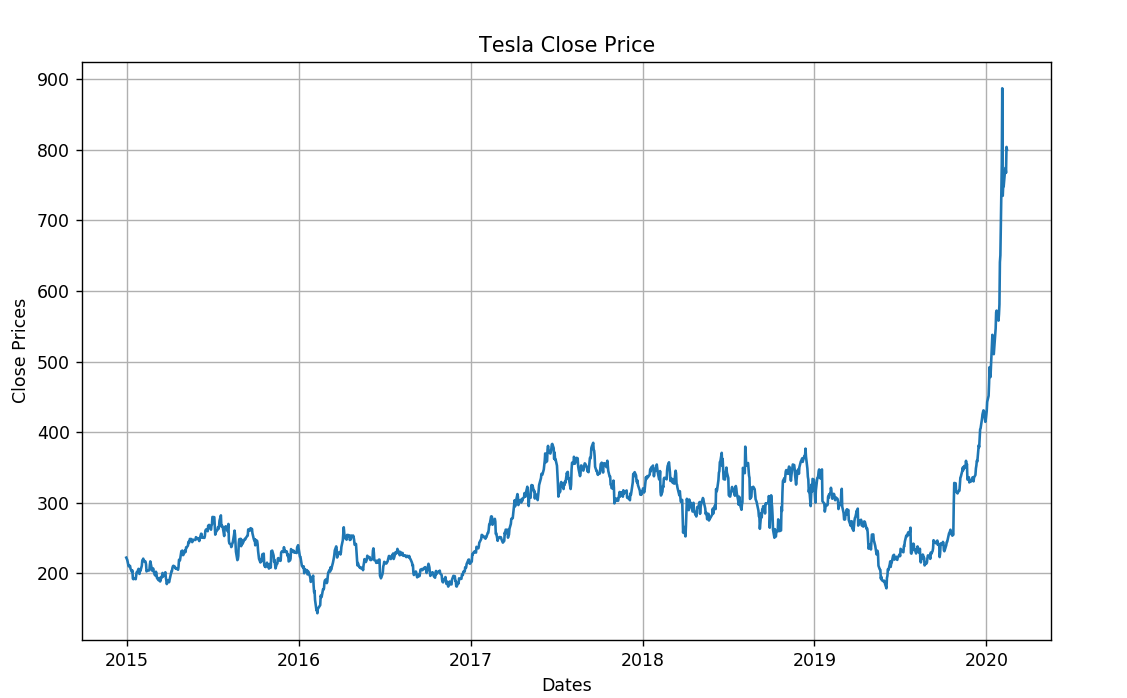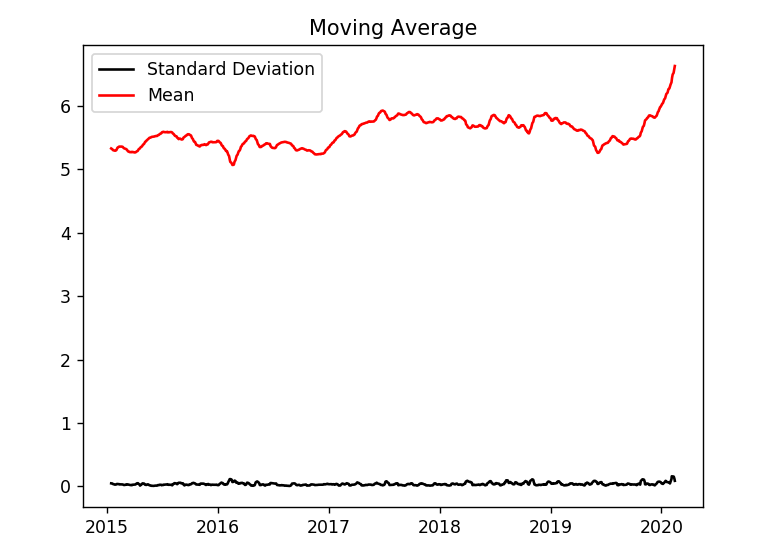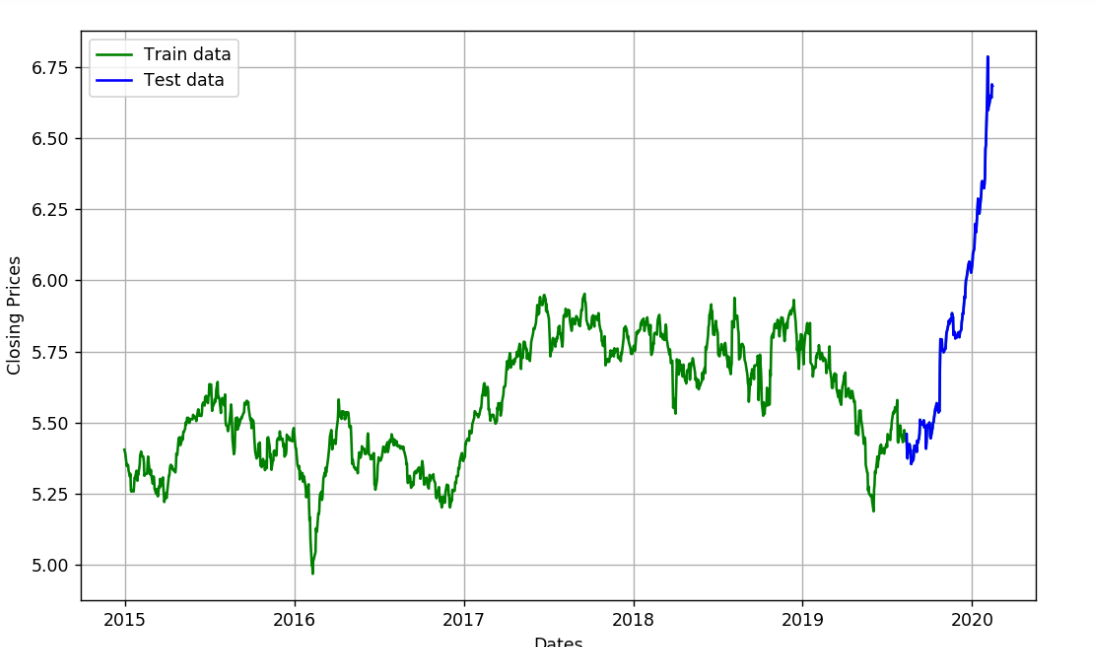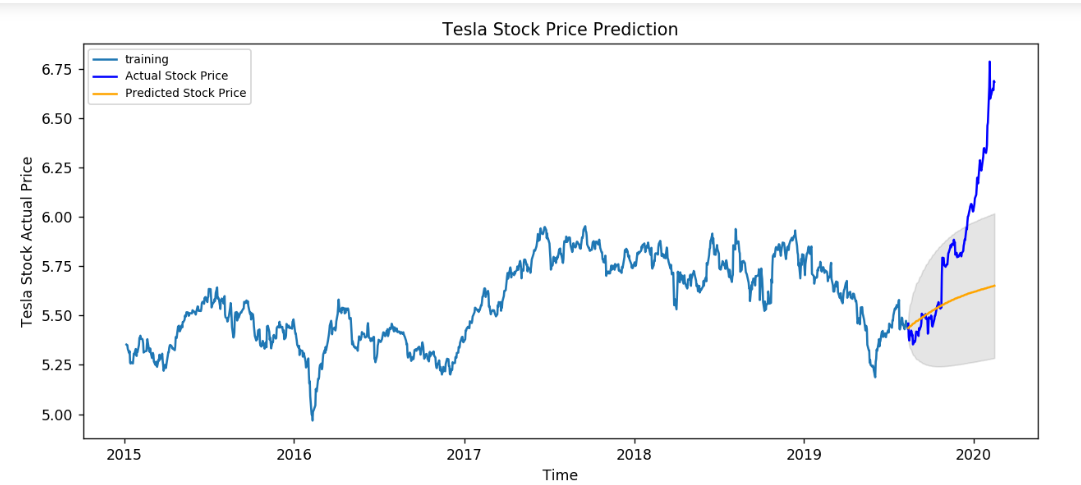1. 从哪里找数据？
2. 如何得到这些数据？

# 步骤

## 获取数据

 ``````1 2 3 4 5 6 `````` ``````import yfinance as yf tlsa_df = yf.download('TSLA', start='2015-01-01', end='2020-02-16', progress=False) tlsa_df.head() ``````

## 绘制价格变化图

 `````` 1 2 3 4 5 6 7 8 9 10 11 `````` ``````#plot %matplotlib notebook from IPython.core.interactiveshell import InteractiveShell InteractiveShell.ast_node_interactivity = "all" plt.figure(figsize=(10,6)) plt.grid(True) plt.xlabel('Dates') plt.ylabel('Close Prices') plt.plot(tlsa_df['Close']) plt.title('Tesla Close Price') plt.show() ``````## 分析数据的统计特征信息

 ``````1 2 3 4 5 6 7 8 9 `````` ``````tsla_df_close = tlsa_df['Close'] df_log = np.log(tsla_df_close) moving_avg = df_log.rolling(12).mean() std_dev = df_log.rolling(12).std() plt.title('Moving Average') plt.plot(std_dev, color ="black", label = "Standard Deviation") plt.plot(moving_avg, color="red", label = "Mean") plt.show() ``````## 处理数据

 ``````1 2 3 4 5 6 7 8 `````` ``````train_data, test_data = df_log[3:int(len(df_log)*0.9)], df_log[int(len(df_log)*0.9):] plt.figure(figsize=(10,6)) plt.grid(True) plt.xlabel('Dates') plt.ylabel('Closing Prices') plt.plot(df_log, 'green', label='Train data') plt.plot(test_data, 'blue', label='Test data') plt.legend() ``````## 建立模型

 `````` 1 2 3 4 5 6 7 8 9 10 11 12 13 14 15 16 17 18 19 20 21 22 23 24 25 26 `````` ``````import os import numpy as np import pandas as pd import matplotlib.pyplot as plt from statsmodels.tsa.stattools import adfuller from statsmodels.tsa.seasonal import seasonal_decompose from statsmodels.tsa.arima_model import ARIMA from pmdarima.arima import auto_arima from sklearn.metrics import mean_squared_error, mean_absolute_error import math model_autoARIMA = auto_arima(train_data, start_p=0, start_q=0, test='adf', # use adftest to find optimal 'd' max_p=3, max_q=3, # maximum p and q m=1, # frequency of series d=None, # let model determine 'd' seasonal=False, # No Seasonality start_P=0, D=0, trace=True, error_action='ignore', suppress_warnings=True, stepwise=True) print(model_autoARIMA.summary()) ``````

 `````` 1 2 3 4 5 6 7 8 9 10 11 12 13 14 15 16 17 18 19 20 21 22 23 24 25 26 `````` ``````Performing stepwise search to minimize aic Fit ARIMA: (0, 1, 0)x(0, 0, 0, 0) (constant=True); AIC=-4980.147, BIC=-4970.040, Time=2.357 seconds Fit ARIMA: (1, 1, 0)x(0, 0, 0, 0) (constant=True); AIC=-4978.315, BIC=-4963.154, Time=0.297 seconds Fit ARIMA: (0, 1, 1)x(0, 0, 0, 0) (constant=True); AIC=-4978.308, BIC=-4963.147, Time=0.475 seconds Fit ARIMA: (0, 1, 0)x(0, 0, 0, 0) (constant=False); AIC=-4982.140, BIC=-4977.087, Time=0.189 seconds Fit ARIMA: (1, 1, 1)x(0, 0, 0, 0) (constant=True); AIC=-4976.958, BIC=-4956.743, Time=0.493 seconds Total fit time: 5.619 seconds SARIMAX Results ============================================================================== Dep. Variable: y No. Observations: 1158 Model: SARIMAX(0, 1, 0) Log Likelihood 2492.070 Date: Mon, 17 Feb 2020 AIC -4982.140 Time: 20:29:52 BIC -4977.087 Sample: 0 HQIC -4980.233 - 1158 Covariance Type: opg ============================================================================== coef std err z P>|z| [0.025 0.975] ------------------------------------------------------------------------------ sigma2 0.0008 1.84e-05 42.731 0.000 0.001 0.001 =============================================================================== Ljung-Box (Q): 43.17 Jarque-Bera (JB): 898.42 Prob(Q): 0.34 Prob(JB): 0.00 Heteroskedasticity (H): 1.84 Skew: -0.13 Prob(H) (two-sided): 0.00 Kurtosis: 7.31 =============================================================================== ``````

## 对模型诊断

 ``````1 2 3 4 5 `````` ``````model_autoARIMA.plot_diagnostics(figsize=(15,8)) plt.show() model = ARIMA(train_data, order=(3, 1, 2)) fitted = model.fit(disp=-1) print(fitted.summary()) ``````

 `````` 1 2 3 4 5 6 7 8 9 10 11 12 13 14 15 16 17 18 19 20 21 22 23 24 25 26 27 28 `````` `````` ARIMA Model Results ============================================================================== Dep. Variable: D.Close No. Observations: 1157 Model: ARIMA(3, 1, 2) Log Likelihood 2494.608 Method: css-mle S.D. of innovations 0.028 Date: Mon, 17 Feb 2020 AIC -4975.215 Time: 20:30:59 BIC -4939.840 Sample: 1 HQIC -4961.866 ================================================================================= coef std err z P>|z| [0.025 0.975] --------------------------------------------------------------------------------- const 0.0002 0.000 1.274 0.203 -0.000 0.001 ar.L1.D.Close 0.1523 0.251 0.607 0.544 -0.339 0.644 ar.L2.D.Close 0.8380 0.235 3.562 0.000 0.377 1.299 ar.L3.D.Close -0.0097 0.032 -0.305 0.760 -0.072 0.053 ma.L1.D.Close -0.1689 0.249 -0.678 0.498 -0.657 0.320 ma.L2.D.Close -0.8311 0.249 -3.334 0.001 -1.320 -0.343 Roots ============================================================================= Real Imaginary Modulus Frequency ----------------------------------------------------------------------------- AR.1 1.0107 +0.0000j 1.0107 0.0000 AR.2 -1.1784 +0.0000j 1.1784 0.5000 AR.3 86.4971 +0.0000j 86.4971 0.0000 MA.1 1.0000 +0.0000j 1.0000 0.0000 MA.2 -1.2033 +0.0000j 1.2033 0.5000 ----------------------------------------------------------------------------- ``````

## 利用模型进行预测

 `````` 1 2 3 4 5 6 7 8 9 10 11 12 13 14 15 `````` ``````fc, se, conf = fitted.forecast(129, alpha=0.05) fc_series = pd.Series(fc, index=test_data.index) lower_series = pd.Series(conf[:, 0], index=test_data.index) upper_series = pd.Series(conf[:, 1], index=test_data.index) plt.figure(figsize=(12,5), dpi=100) plt.plot(train_data, label='training') plt.plot(test_data, color = 'blue', label='Actual Stock Price') plt.plot(fc_series, color = 'orange',label='Predicted Stock Price') plt.fill_between(lower_series.index, lower_series, upper_series, color='k', alpha=.10) plt.title('Tesla Stock Price Prediction') plt.xlabel('Time') plt.ylabel('Tesla Stock Price') plt.legend(loc='upper left', fontsize=8) plt.show() ``````## 分析预测结果

1. 预测结果与实际股票的上涨趋势相同，但是其中的路径还是相差很多，直接说明不适合指导具体交易，只能做为长期趋势判断的参考
2. 这个模型还需要大量进行测试，如调整数据，调整模型参数

# 总结

1. 本文只是进行一个过程展示
2. 本文中的模型只供参考，不能用于实际的股票投资与交易。股市有风险，入市当小心。

# 欢迎关注